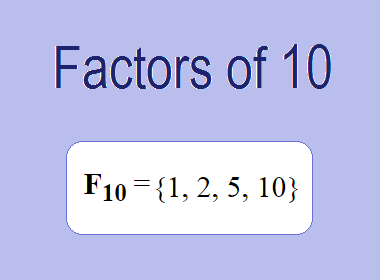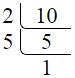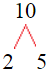# Factors of 10The factors of 10 are 1, 2, 5, and 10 i.e. F10 = {1, 2, 5, 10}. The factors of 10 are all the numbers that can divide 10 without leaving a remainder.

We can check if these numbers are factors of 10 by dividing 10 by each of them. If the result is a whole number, then the number is a factor of 10. Let's do this for each of the numbers listed above:

·        1 is a factor of 10 because 10 divided by 1 is 10.

·        2 is a factor of 10 because 10 divided by 2 is 5.

·        5 is a factor of 10 because 10 divided by 5 is 2.

·        10 is a factor of 10 because 10 divided by 10 is 1.

## How to Find Factors of 10?

1 and the number itself are the factors of every number. So, 1 and 10 are two factors of 10. To find the other factors of 10, we can start by dividing 10 by the numbers between 1 and 10. If we divide 10 by 2, we get a remainder of 0. Therefore, 2 is a factor of 10. If we divide 10 by 3, we get a remainder of 1. Therefore, 3 is not a factor of 10.

Next, we can check if 4 is a factor of 10. If we divide 10 by 4, we get a remainder of 2. Therefore, 4 is not a factor of 10. We can continue this process for all the possible factors of 10.

Through this process, we can find that the factors of 10 are 1, 2, 5, and 10. These are the only numbers that can divide 10 without leaving a remainder.

********************

********************

## Properties of the Factors of 10

The factors of 10 have some interesting properties. One of the properties is that the sum of the factors of 10 is equal to 18. We can see this by adding all the factors of 10 together:

1 + 2 + 5 + 10 = 18

Another property of the factors of 10 is that the prime factors of 10 are 2 and 5 only.

## Applications of the Factors of 10

The factors of 10 have several applications in mathematics. One of the applications is in finding the highest common factor (HCF) of two or more numbers. The HCF is the largest factor that two or more numbers have in common. For example, to find the HCF of 10 and 15, we need to find the factors of both numbers and identify the largest factor they have in common. The factors of 10 are 1, 2, 5, and 10. The factors of 15 are 1, 3, 5, and 15. The largest factor that they have in common is 5. Therefore, the HCF of 10 and 15 is 5.

Another application of the factors of 10 is in prime factorization. Prime factorization is the process of expressing a number as the product of its prime factors. The prime factors of 10 are 2 and 5, since these are the only prime numbers that can divide 10 without leaving a remainder. Therefore, we can express 10 as:

10 = 2 × 5

We can do prime factorization by division and factor tree method also. Here is the prime factorization of 10 by division method,10 = 2 × 5

Here is the prime factorization of 10 by the factor tree method,10 = 2 × 5

## Conclusion

The factors of 10 are the numbers that can divide 10 without leaving a remainder. The factors of 10 are 1, 2, 5, and 10. The factors of 10 have some interesting properties, such as having a sum of 18. The factors of 10 have several applications in mathematics, such as finding the highest common factor and prime factorization.